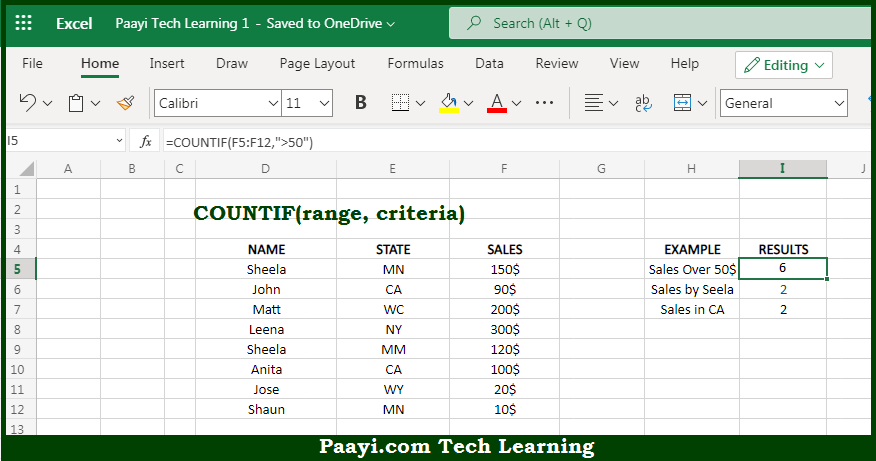# Learn How to Use Microsoft Excel COUNTIF Function

Written by | 0 Comments | 422 Views

In this article, you will learn how to use the Microsoft Excel COUNTIF function and its prime function in Microsoft Excel. You will also get to know the Microsoft Excel COUNTIF function return value and syntax with the help of some examples.

Microsoft Excel COUNTIF Function

The main purpose of the Microsoft Excel COUNTIF function is to count the cells that match the criteria. That implies, with the help of the COUNTIF function you can able to return a count of a cell that matches a single condition. It should be noted that the COUNTIF function can be used to count cells that contain dates, numbers, and text. The criteria used in COUNTIF supports logical operators (>,<,<>,=) and wildcards (*,?) for partial matching. So, with the help of the COUNTIF function, you can able to count the cells that match the criteria.

Return Value of COUNTIF Function

The return value will be the number representing the cells counted.

Syntax of COUNTIF Function

=COUNTIF(range, criteria)

Where the arguments:

• range: This is the range of cells to count.
• criteria: These are criteria to decide which cell should be counted.

## How to Use Microsoft Excel COUNTIF Function?So we know that Microsoft Excel COUNTIF function you can able to count the cells that match the criteria. That implies, with the help of the COUNTIF function you can able to return a count of a cell that matches a single condition. It should be noted that the COUNTIF function can be used to count cells that contain dates, numbers, and text.

The criteria used in COUNTIF supports logical operators (>,<,<>,=) and wildcards (*,?) for partial matching. So, with the help of the COUNTIF function, you can able to count the cells that match the criteria.